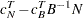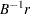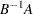The LP Procedure
 Output Data Sets

The LP procedure can optionally produce five output data sets. These are the ACTIVEOUT=, PRIMALOUT=, DUALOUT=, TABLEAUOUT=, and MPSOUT= data sets. Each contains two variables that identify the particular problem in the input data set. These variables are

_OBJ_ID_

identifies the objective function ID.

_RHS_ID_

identifies the right-hand-side variable.

Additionally, each data set contains other variables, which are discussed below.

ACTIVEOUT= Data Set

The ACTIVEOUT= data set contains a representation of the current active branch-and-bound tree. You can use this data set to initialize the branch-and-bound tree to continue iterations on an incompletely solved problem. Each active node in the tree generates two observations in this data set. The first is a 'LOWERBD' observation that is used to reconstruct the lower-bound constraints on the currently described active node. The second is an 'UPPERBD' observation that is used to reconstruct the upper-bound constraints on the currently described active node. In addition to these, an observation that describes the current best integer solution is included. The data set contains the following variables:

_STATUS_

contains the keywords LOWERBD, UPPERBD, and INTBEST for identifying the type of observation.

_PROB_

contains the problem number for the current observation.

_OBJECT_

contains the objective value of the parent problem that generated the current observation’s problem.

_SINFEA_

contains the sum of the integer infeasibilities of the current observation’s problem.

_PROJEC_

contains the data needed for CANSELECT=PROJECT when the branch-and-bound tree is read using the ACTIVEIN= option.

_PSEUDO_

contains the data needed for CANSELECT=PSEUDOC when the branch-and-bound tree is read using the ACTIVEIN= option.

INTEGER VARIABLES

Integer-constrained structural variables are also included in the ACTIVEOUT= data set. For each observation, these variables contain values for defining the active node in the branch-and-bound tree.

PRIMALOUT= Data Set

The PRIMALOUT= data set contains the current primal solution. If the problem has integer-constrained variables, the PRIMALOUT= data set contains the current best integer feasible solution. If none have been found, the PRIMALOUT= data set contains the relaxed solution. In addition to _OBJ_ID_ and _RHS_ID_, the data set contains the following variables:

_VAR_

identifies the variable name.

_TYPE_

identifies the type of the variable as specified in the input data set. Artificial variables are labeled as type 'ARTIFCL'.

_STATUS_

identifies whether the variable is basic, nonbasic, or at an upper bound in the current solution.

_LBOUND_

contains the input lower bound on the variable unless the variable is integer-constrained and an integer solution is given. In this case, _LBOUND_ contains the lower bound on the variable needed to realize the integer solution on subsequent calls to PROC LP when using the PRIMALIN= option.

_VALUE_

identifies the value of the variable in the current solution or the current best integer feasible solution.

_UBOUND_

contains the input upper bound on the variable unless the variable is integer-constrained and an integer solution is given. In this case, _UBOUND_ contains the upper bound on the variable needed to realize the integer solution on subsequent calls to PROC LP when using the PRIMALIN= option.

_PRICE_

contains the input price coefficient of the variable.

_R_COST_

identifies the value of the reduced cost in the current solution. Example 5.3 in the section Examples: LP Procedure shows a typical PRIMALOUT= data set. Note that it is necessary to include the information on objective function and right-hand side in order to distinguish problems in multiple problem data sets.

DUALOUT= Data Set

The DUALOUT= data set contains the dual solution for the current solution. If the problem has integer-constrained variables, the DUALOUT= data set contains the dual for the current best integer solution, if any. Otherwise it contains the dual for the relaxed solution. In addition to _OBJ_ID_ and _RHS_ID_, it contains the following variables:

_ROW_ID_

identifies the row or constraint name.

_TYPE_

identifies the type of the row as specified in the input data set.

_RHS_

gives the value of the right-hand side on input.

_L_RHS_

gives the lower bound for the row evaluated from the input right-hand-side value, the TYPE of the row, and the value of the RANGE variable for the row.

_VALUE_

gives the value of the row, at optimality, excluding logical variables.

_U_RHS_

gives the upper bound for the row evaluated from the input right-hand-side value, the TYPE of the row, and the value of the RANGE variable for the row.

_DUAL_

gives the value of the dual variable associated with the row.

TABLEAUOUT= Data Set

The TABLEAUOUT= data set contains the current tableau. Each observation, except for the first, corresponds to a basic variable in the solution. The observation labeled R_COSTS contains the reduced costs. In addition to _OBJ_ID_ and _RHS_ID_, it contains the following variables:

_BASIC_

gives the names of the basic variables in the solution.

INVB_R

gives the values of, whereis the right-hand-side vector.

STRUCTURAL VARIABLES

give the values in the tableau, namely.

MPSOUT= Data Set

The MPSOUT= data set contains problem data converted from a PROC LP format into an MPS-format SAS data set. The six fields, FIELD1 to FIELD6, in the MPSOUT= data set correspond to the six columns in MPS standard. For more information about the MPS-format SAS data set, see Chapter 16, The MPS-Format SAS Data Set.Previous Page | Next Page | Top of Page